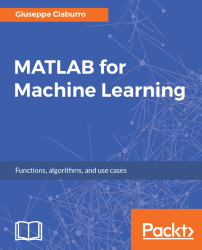•#### MATLAB for Machine Learning#### Overview of this book

MATLAB is the language of choice for many researchers and mathematics experts for machine learning. This book will help you build a foundation in machine learning using MATLAB for beginners. You’ll start by getting your system ready with t he MATLAB environment for machine learning and you’ll see how to easily interact with the Matlab workspace. We’ll then move on to data cleansing, mining and analyzing various data types in machine learning and you’ll see how to display data values on a plot. Next, you’ll get to know about the different types of regression techniques and how to apply them to your data using the MATLAB functions. You’ll understand the basic concepts of neural networks and perform data fitting, pattern recognition, and clustering analysis. Finally, you’ll explore feature selection and extraction techniques for dimensionality reduction for performance improvement. At the end of the book, you will learn to put it all together into real-world cases covering major machine learning algorithms and be comfortable in performing machine learning with MATLAB.
Title PageCreditsForewordwww.PacktPub.comCustomer FeedbackPrefaceFree Chapter
Getting Started with MATLAB Machine LearningImporting and Organizing Data in MATLABFrom Data to Knowledge DiscoveryFinding Relationships between Variables - Regression TechniquesPattern Recognition through Classification AlgorithmsIdentifying Groups of Data Using Clustering MethodsSimulation of Human Thinking - Artificial Neural NetworksImproving the Performance of the Machine Learning Model - Dimensionality ReductionMachine Learning in Practice## Partitioning around the actual center - K-medoids clustering

K-medoids is a partitioning clustering algorithm related to the K-means algorithm. Given a set of n objects and a k number that determines how many clusters you want to output, K-medoids divides the dataset into groups, trying to minimize the average quadratic error, the distance between the points of a cluster, and the point designated to be the center.

### What is a medoid?

Unlike K-means, where this point is artificial (that is, the pure average of all points in the cluster also named centroid), in K-medoids, the point used is placed more centrally; so the center is one of the actual data points (medoid). A medoid can be defined as an object of a cluster whose average disparity over all objects in the cluster is minimal; thus, it will be the most central point of a given dataset. K-medoids are more robust to noise and outliers than K-means, because a mean is easily influenced by extreme values.

### The kmedoids() function

In MATLAB, K-medoids...